# Selina Concise Chemistry Solution for ICSE Class 10 Chapter 4 Analytical Chemistry: Uses of Ammonium Hydroxide and Sodium Hydroxide

Analytical chemistry is a part of chemistry that mainly deals with the analysis of compounds. Thus, studying chapter 4 will require students to familiarize themselves with colours of some important salts and their solutions while understanding the action of Ammonium Hydroxide or Sodium Hydroxide on certain salts. While these are the main objectives of the chapter, concise Chemistry class 10 ICSE Solutions for chapter 4 that is given here will help students get a clear insight into the important concepts and topics. These solutions include detailed explanations and accurate answers to all the questions given at the end of the chapter. Students can, therefore, use these solutions extensively to complete assignments, study productively and score better marks in the board examinations.

## Selina Concise Chemistry Solution Class 10 Chapter 4 Analytical Chemistry: Uses of Ammonium Hydroxide and Sodium Hydroxide:-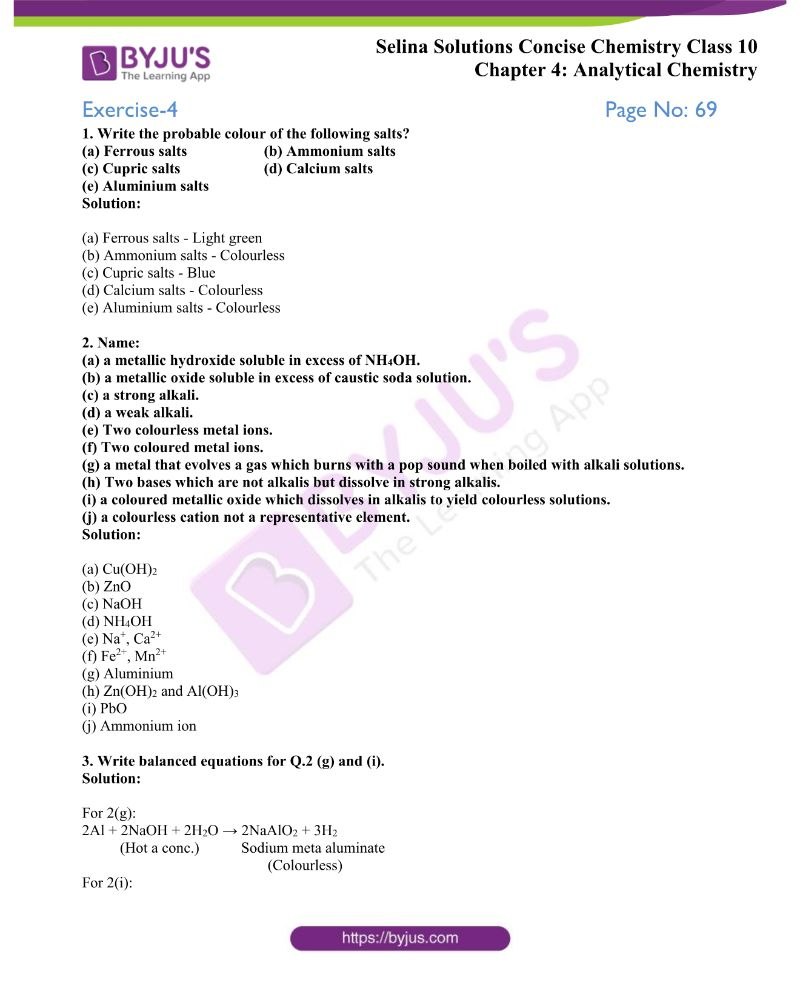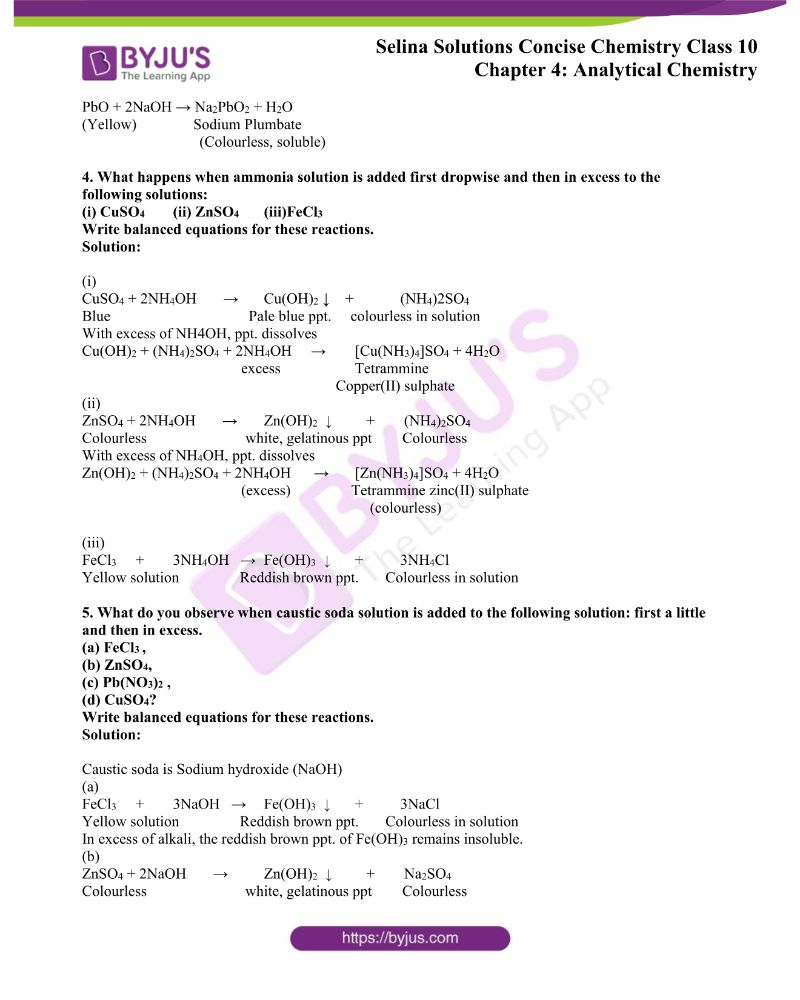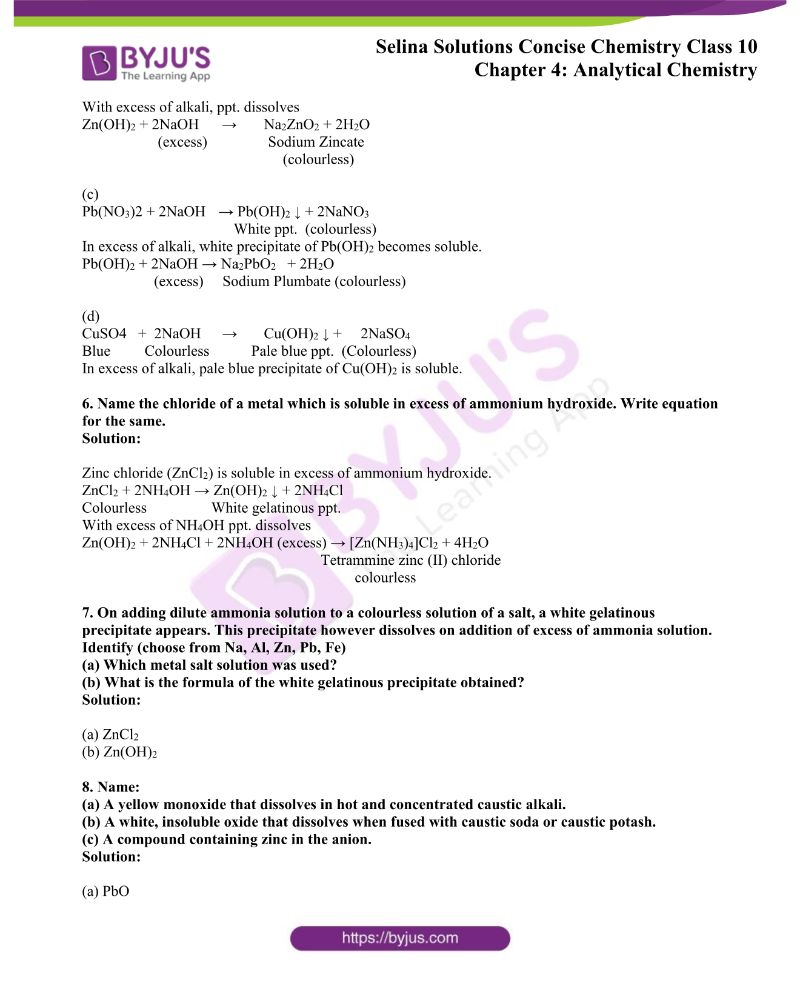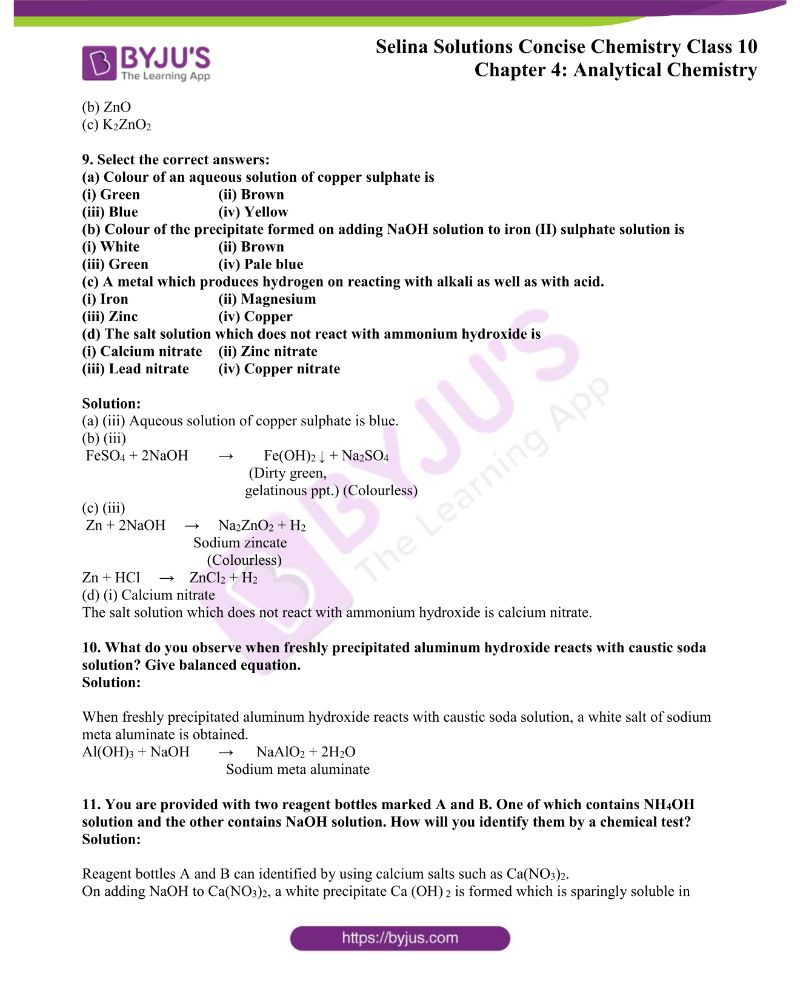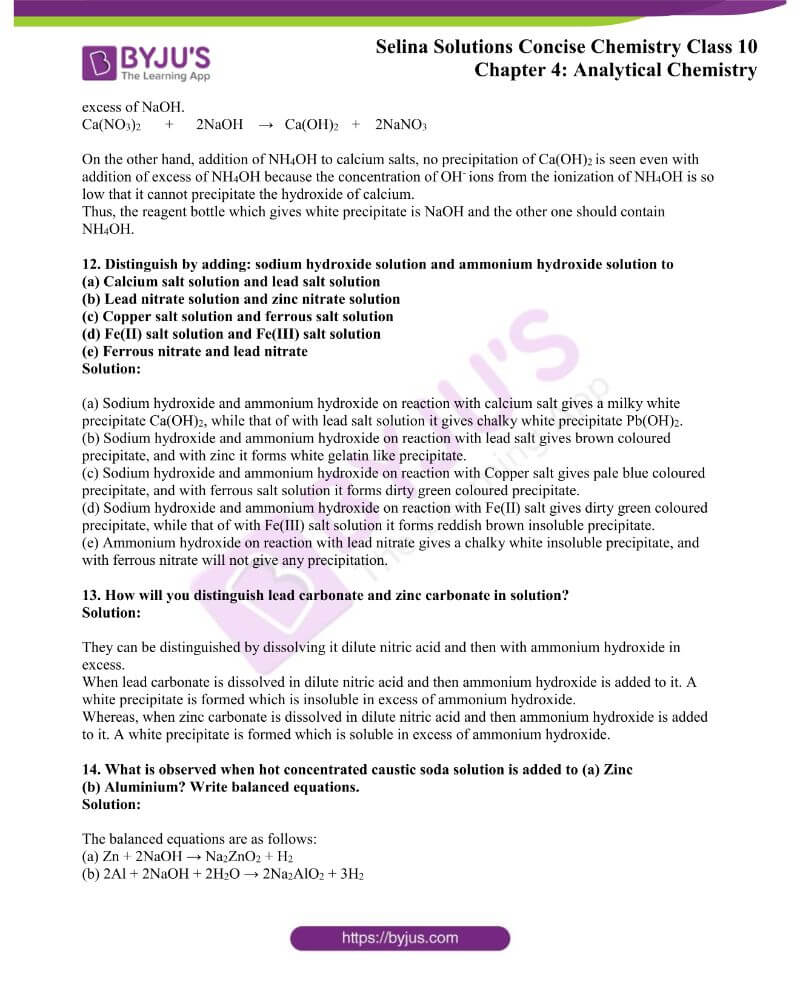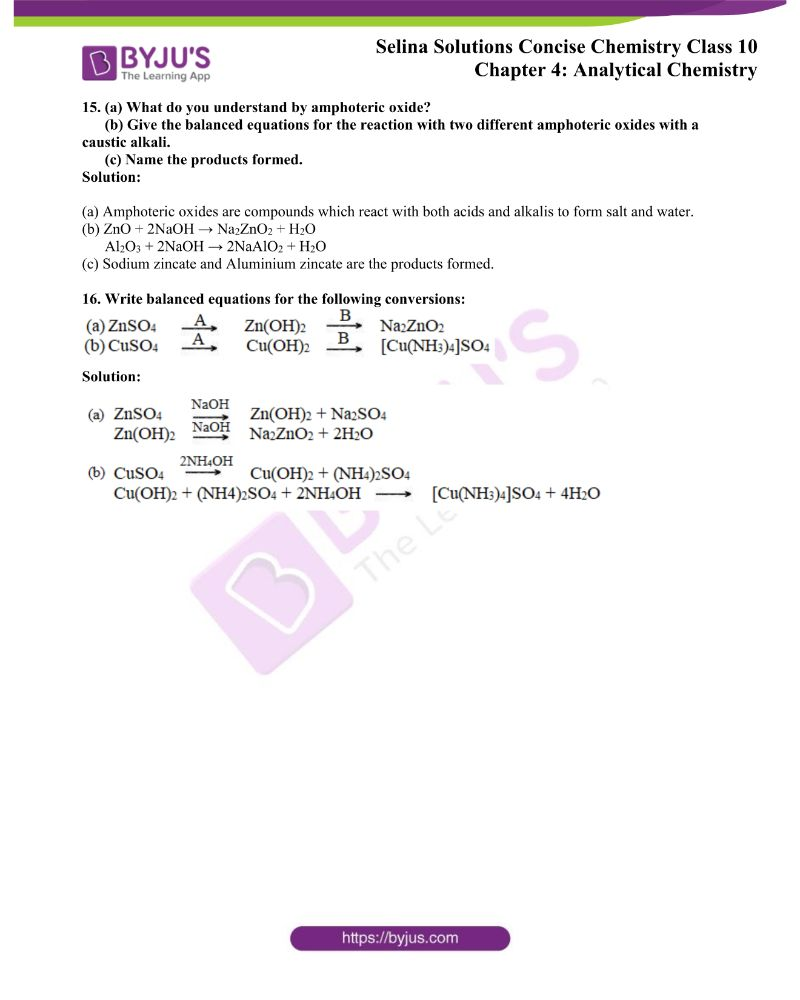### Access ICSE Selina Class 10 Chapter 4 Analytical Chemistry: Uses of Ammonium Hydroxide and Sodium Hydroxide Solutions

Exercise-4 Page No: 69

1. Write the probable colour of the following salts?

(a) Ferrous salts (b) Ammonium salts

(c) Cupric salts (d) Calcium salts

(e) Aluminium salts

Solution:

(a) Ferrous salts – Light green

(b) Ammonium salts – Colourless

(c) Cupric salts – Blue

(d) Calcium salts – Colourless

(e) Aluminium salts – Colourless

2. Name:

(a) a metallic hydroxide soluble in excess of NH4OH.

(b) a metallic oxide soluble in excess of caustic soda solution.

(c) a strong alkali.

(d) a weak alkali.

(e) Two colourless metal ions.

(f) Two coloured metal ions.

(g) a metal that evolves a gas which burns with a pop sound when boiled with alkali solutions.

(h) Two bases which are not alkalis but dissolve in strong alkalis.

(i) a coloured metallic oxide which dissolves in alkalis to yield colourless solutions.

(j) a colourless cation, not a representative element.

Solution:

(a) Cu(OH)2

(b) ZnO

(c) NaOH

(d) NH4OH

(e) Na+, Ca2+

(f) Fe2+, Mn2+

(g) Aluminium

(h) Zn(OH)2 and Al(OH)3

(i) PbO

(j) Ammonium ion

3. Write balanced equations for Q.2 (g) and (i).

Solution:

For 2(g):

2Al + 2NaOH + 2H2O → 2NaAlO2 + 3H2

(Hot a conc.) Sodium meta aluminate

(Colourless)

For 2(i):

PbO + 2NaOH → Na2PbO2 + H2O

(Yellow) Sodium Plumbate

(Colourless, soluble)

4. What happens when ammonia solution is added first dropwise and then in excess to the following solutions:

(i) CuSO4 (ii) ZnSO4 (iii)FeCl3

Write balanced equations for these reactions.

Solution:

(i)

CuSO4 + 2NH4OH → Cu(OH)2 ↓ + (NH4)2SO4

Blue Pale blue ppt. colourless in solution

With an excess of NH4OH, ppt. dissolves

Cu(OH)2 + (NH4)2SO4 + 2NH4OH → [Cu(NH3)4]SO4 + 4H2O

excess Tetrammine

Copper(II) sulphate

(ii)

ZnSO4 + 2NH4OH → Zn(OH)2 ↓ + (NH4)2SO4

Colourless white, gelatinous ppt Colourless

With an excess of NH4OH, ppt. dissolves

Zn(OH)2 + (NH4)2SO4 + 2NH4OH → [Zn(NH3)4]SO4 + 4H2O

(excess) Tetrammine zinc(II) sulphate

(colourless)

(iii)

FeCl3 + 3NH4OH → Fe(OH)3 ↓ + 3NH4Cl

Yellow solution Reddish-brown ppt. Colourless in solution

5. What do you observe when caustic soda solution is added to the following solution: first a little and then in excess.

(a) FeCl,

(b) ZnSO4,

(c) Pb(NO3)2,

(d) CuSO4?

Write balanced equations for these reactions.

Solution:

Caustic soda is Sodium hydroxide (NaOH)

(a)

FeCl3 + 3NaOH → Fe(OH)3 ↓ + 3NaCl

Yellow solution Reddish-brown ppt. Colourless in solution

In excess of alkali, the reddish-brown ppt. of Fe(OH)3 remains insoluble.

(b)

ZnSO4 + 2NaOH → Zn(OH)2 ↓ + Na2SO4

Colourless white, gelatinous ppt Colourless

With an excess of alkali, ppt. dissolves

Zn(OH)2 + 2NaOH → Na2ZnO2 + 2H2O

(excess) Sodium Zincate

(colourless)

(c)

Pb(NO3)2 + 2NaOH → Pb(OH)2 ↓ + 2NaNO3

White ppt. (colourless)

In excess of alkali, white precipitate of Pb(OH)2 becomes soluble.

Pb(OH)2 + 2NaOH → Na2PbO2 + 2H2O

(excess) Sodium Plumbate (colourless)

(d)

CuSO4 + 2NaOH → Cu(OH)2 ↓ + 2NaSO4

Blue Colourless Pale blue ppt. (Colourless)

In excess of alkali, pale blue precipitate of Cu(OH)2 is soluble.

6. Name the chloride of a metal which is soluble in excess of ammonium hydroxide. Write equation for the same.

Solution:

Zinc chloride (ZnCl2) is soluble in excess of ammonium hydroxide.

ZnCl2 + 2NH4OH → Zn(OH)2 ↓ + 2NH4Cl

Colourless White gelatinous ppt.

With an excess of NH4OH ppt. dissolves

Zn(OH)2 + 2NH4Cl + 2NH4OH (excess) → [Zn(NH3)4]Cl2 + 4H2O

Tetrammine zinc (II) chloride

colourless

7. On adding dilute ammonia solution to a colourless solution of a salt, a white gelatinous precipitate appears. This precipitate, however, dissolves on addition of an excess of ammonia solution. Identity (choose from Na, Al, Zn, Pb, Fe)

(a) Which metal salt solution was used?

(b) What is the formula of the white gelatinous precipitate obtained?

Solution:

(a) ZnCl2

(b) Zn(OH)2

8. Name:

(a) A yellow monoxide that dissolves in hot and concentrated caustic alkali.

(b) A white, insoluble oxide that dissolves when fused with caustic soda or caustic potash.

(c) A compound containing zinc in the anion.

Solution:

(a) PbO

(b) ZnO

(c) K2ZnO2

(a) Colour of an aqueous solution of copper sulphate is

(i) Green (ii) Brown

(iii) Blue (iv) Yellow

(b) Colour of the precipitate formed on adding NaOH solution to iron (II) sulphate solution is

(i) White (ii) Brown

(iii) Green (iv) Pale blue

(c) A metal which produces hydrogen on reacting with alkali as well as with acid.

(i) Iron (ii) Magnesium

(iii) Zinc (iv) Copper

(d) The salt solution which does not react with ammonium hydroxide is

(i) Calcium nitrate (ii) Zinc nitrate

(iii) Lead nitrate (iv) Copper nitrate

Solution:

(a) (iii) An aqueous solution of copper sulphate is blue.

(b) (iii)

FeSO4 + 2NaOH  →  Fe(OH)↓ + Na2SO4

(Dirty green,

gelatinous ppt.) (Colourless)

(c) (iii)

Zn + 2NaOH  →  Na2ZnO2 + H2

Sodium zincate

(Colourless)

Zn + HCl  → ZnCl2 + H2

(d) (i) Calcium nitrate

The salt solution which does not react with ammonium hydroxide is calcium nitrate.

10. What do you observe when freshly precipitated aluminum hydroxide reacts with caustic soda solution? Give a balanced equation.

Solution:

When freshly precipitated aluminum hydroxide reacts with caustic soda solution, a white salt of sodium meta aluminate is obtained.

Al(OH)3 + NaOH → NaAlO2 + 2H2O

Sodium meta aluminate

11. You are provided with two reagent bottles marked A and B. One of which contains NH4OH solution and the other contains NaOH solution. How will you identify them by a chemical test?

Solution:

Reagent bottles A and B can be identified by using calcium salts such as Ca(NO3)2.

On adding NaOH to Ca(NO3)2, a white precipitate Ca (OH) 2 is formed which is sparingly soluble in excess of NaOH.

Ca(NO3)2 + 2NaOH → Ca(OH)2 + 2NaNO3

On the other hand, the addition of NH4OH to calcium salts, no precipitation of Ca(OH)is seen even with the addition of an excess of NH4OH because the concentration of OHions from the ionization of NH4OH is so low that it cannot precipitate the hydroxide of calcium.

Thus, the reagent bottle which gives white precipitate is NaOH and the other one should contain NH4OH.

12. Distinguish by adding: sodium hydroxide solution and ammonium hydroxide solution to

(a) Calcium salt solution and lead salt solution

(b) Lead nitrate solution and zinc nitrate solution

(c) Copper salt solution and ferrous salt solution

(d) Fe(II) salt solution and Fe(III) salt solution

(e) Ferrous nitrate and lead nitrate

Solution:

(a) Sodium hydroxide and ammonium hydroxide on reaction with calcium salt give a milky white precipitate Ca(OH)2, while that of with lead salt solution it gives chalky white precipitate Pb(OH)2.

(b) Sodium hydroxide and ammonium hydroxide on reaction with lead salt give brown coloured precipitate, and with zinc, it forms a white gelatin-like precipitate.

(c) Sodium hydroxide and ammonium hydroxide on reaction with Copper salt give pale blue coloured precipitate, and with a ferrous salt solution, it forms a dirty green coloured precipitate.

(d) Sodium hydroxide and ammonium hydroxide on reaction with Fe(II) salt give dirty green coloured precipitate, while that of with Fe(III) salt solution it forms a reddish brown insoluble precipitate.

(e) Ammonium hydroxide on reaction with lead nitrate gives a chalky white insoluble precipitate, and with ferrous nitrate will not give any precipitation.

13. How will you distinguish lead carbonate and zinc carbonate in solution?

Solution:

They can be distinguished by dissolving it dilute nitric acid and then with ammonium hydroxide in excess.

When lead carbonate is dissolved in dilute nitric acid and then ammonium hydroxide is added to it. A white precipitate is formed which is insoluble in excess of ammonium hydroxide.

Whereas, when zinc carbonate is dissolved in dilute nitric acid and then ammonium hydroxide is added to it. A white precipitate is formed which is soluble in excess of ammonium hydroxide.

14. What is observed when hot concentrated caustic soda solution is added to (a) Zinc (b) Aluminium? Write balanced equations.

Solution:

The balanced equations are as follows:

(a) Zn + 2NaOH → Na2ZnO2 + H2

(b) 2Al + 2NaOH + 2H2O → 2Na2AlO2 + 3H2

15. (a) What do you understand by amphoteric oxide?

(b) Give the balanced equations for the reaction with two different amphoteric oxides with caustic alkali.

(c) Name the products formed.

Solution:

(a) Amphoteric oxides are compounds which react with both acids and alkalis to form salt and water.

(b) ZnO + 2NaOH → Na2ZnO2 + H2O

Al2O3 + 2NaOH → 2NaAlO2 + H2O

(c) Sodium zincate and Aluminium zincate are the products formed.

16. Write balanced equations for the following conversions: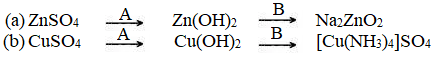Solution: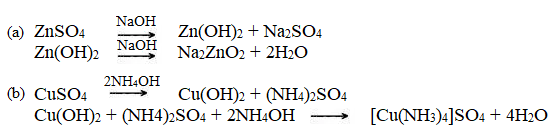The given solutions are as per the 2019-20 Concise Selina textbook. The Selina Solutions for the academic year 2023-24 will be updated soon.